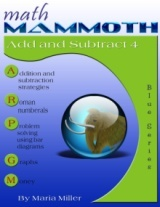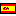^
You are here: HomeBlue Series → Add & Subtract 4

# Math Mammoth Add & Subtract 455 pages

## Prices & ordering

Printed copy \$8.65Este libro en español

Math Mammoth Add & Subtract 4 is a workbook for 4th grade, and it covers the following topics related to addition and subtraction:

• mental addition and subtraction strategies;
• adding and subtracting in columns;
• some numerical patterns;
• bar models in problem solving;
• order of operations;
• line graphs and bar graphs;
• rounding and estimating;
• money problems and discounts.

The PDF version of this book is enabled for annotation. This means that if you prefer, the student can fill it in on the computer, using the typewriter and drawing tools in Adobe Reader version 9 or greater.

The lessons in this book are taken from the first chapter of Math Mammoth Grade 4 Complete Curriculum. Some of the lessons are even useful for 5th grade students, especially if they have not had much exposure to word problems, the order of operations, and applications of addition and subtraction.

At first, we review the "technical aspects" of adding and subtracting: mental math techniques and adding and subtracting in columns. We also study some patterns. The lesson on Pascal's triangle is intended to be fun and fascinating—after all, Pascal's triangle is full of patterns!

In the next lesson, we study the connection between addition and subtraction and bar models. Bar models help students write addition and subtraction sentences with unknowns, and solve them. This is teaching the students algebraic thinking: how to write and solve simple equations.

The lesson on the order of operations contains some review, but we also study connecting the topic with real-life situations (such as shopping). Here, the student writes the mathematical expression (number sentence) for word problems, which again, practices algebraic thinking.

Going towards applications of math, the book then contains straightforward lessons on bar graphs, line graphs, rounding, estimating, and money problems.

You might also be interested in:
• Math Mammoth Add & Subtract 3—a worktext about adding and subtracting 3-digit numbers with regrouping and with mental math, estimation, and word problems (grades 2-3).
• Math Mammoth Place Value 4—a worktext about thousands, ten thousands, and hundred thousands, addition and subtraction with those numbers, comparing, and the idea of multiples (grades 4-5).
• Make It Real Learning: Arithmetic I—an activity workbook with real-world data, focusing on situations that use addition, subtraction, estimation, division, bar graphs, and place value with large numbers (grades 3-6).

## Better Yet - Bundle Deals!

*BONUS*: Buy any bundle listed above, and get the fully upgraded Soft-Pak software for FREE (includes 4 math and 2 language arts programs, complete with on screen and printable options).

## How and where to order

You can buy Math Mammoth books at:

• Here at MathMammoth.com website — simply use the "Add to cart" buttons you see on the product pages.
• Rainbow Resource carries printed copies for the Light Blue series books, plus several CDs (Light Blue and Blue series).
• At Teachers Pay Teachers you can purchase the Light Blue Series downloads, plus topical units.
• Lulu sells printed copies for most of the Math Mammoth materials (various series).

By purchasing any of the books, permission IS granted for the teacher (or parent) to reproduce this material to be used with his/her students in a teaching situation; not for commercial resale. However, you are not permitted to share the material with another teacher.

In other words, you are permitted to make copies for the students/children you are teaching, but not for other teachers' usage.

Math Mammoth books are PDF files. You will need Adobe Reader to view them, including if you use a Mac or Linux. You can try other PDF viewers, but they seem to either omit or mess up some of the images.
WAIT!

Receive my monthly collection of math tips & resources directly in your inbox — and get a FREE Math Mammoth book!You can unsubscribe at any time.

### Math Mammoth Tour

Confused about the different options? Take a virtual email tour around Math Mammoth! You'll receive:

An initial email to download your GIFT of over 400 free worksheets and sample pages from my books. Six other "TOURSTOP" emails that explain the important things and commonly asked questions concerning Math Mammoth curriculum. (Find out the differences between all these different-colored series!)

This way, you'll have time to digest the information over one or two weeks, plus an opportunity to ask me personally about the curriculum.
A monthly collection of math teaching tips & Math Mammoth updates (unsubscribe any time)### "Mini" Math Teaching Course

This is a little "virtual" 2-week course, where you will receive emails on important topics on teaching math, including:

- How to help a student who is behind
- Troubles with word problems
- Teaching multiplication tables
- Why fractions are so difficult
- The value of mistakes
- Should you use timed tests
- And more!

A GIFT of over 400 free worksheets and sample pages from my books right in the very beginning.A monthly collection of math teaching tips & Math Mammoth updates (unsubscribe any time)
Enter your email to receive math teaching tips, resources, Math Mammoth news & sales, humor, and more! I tend to send out these tips about once monthly, near the beginning of the month, but occasionally you may hear from me twice per month (and sometimes less often).• A GIFT of over 400 free worksheets and sample pages from my books.